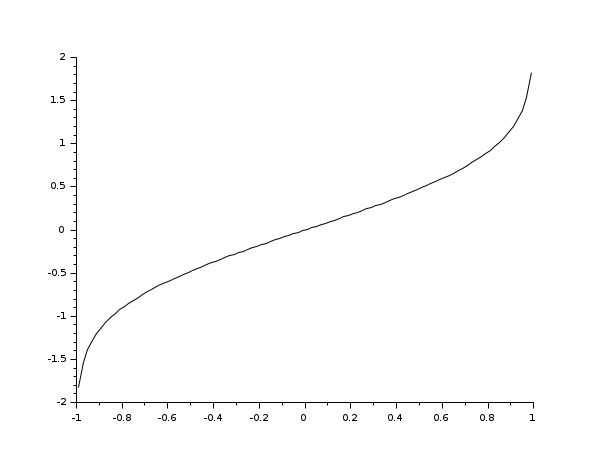# erfinv

inverse error function

### Syntax

`y = erfinv(x)`

### Arguments

x

vector or matrix

y

vector or matrix (same size as x)

### Description

The `erfinv` function computes the inverse of the `erf` error function. Thus, erf(erfinv(x)) = x for all `x` such that ```-1 ≤ x ≤ 1``` .

### Examples

```x = linspace(-0.99, 0.99, 100);
y = erfinv(x);
plot2d(x, y)```